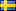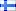# DC-DC converter

## DC to DC converter

A buck converter (step-down converter) is used when the output voltage (VDC) needs to be lower than on the input voltage (VDC).

A boost converter (step-up converter) is used when the output voltage (VDC) needs to be higher than the input voltage.

A buck-boost converter (step-down/step-up converter) buck-boost converter is a type of DC-to-DC converter that has an output voltage that is either greater than or less than the input voltage

A voltage stabilizer (step-down/step-up) is used when the output voltage (VDC) should be constant but the input voltage (VDC) varies with above or below the output voltage (VDC)

A step-down converter is used when the output voltage (VDC) needs to be lower than on the input voltage (VDC).

Produced by: Wikinggruppen
##### Customer's Area